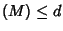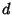## Cheeger's Finiteness Theorem

Consider the set of compact-Riemannian Manifoldswith diameter, Volume, andwhereis the Sectional Curvature. Then there is a bound on the number of Diffeomorphisms classes of this set in terms of the constants,,, and.

References

Chavel, I. Riemannian Geometry: A Modern Introduction. New York: Cambridge University Press, 1994.